M-Set Derivatives

One way of coloring the inside of the M-set is to work with the the various derivatives that describe the 'flow' of Zn. These images are all based on the computation of the derivative of Zn with respect to c. The expression for this is simple: Z'n+1 = dZn+1/dc = 2 * Zn * Z'n + 1, which must be iterated. Note that this derivative can be though of as a vector: it has a real component and an imaginary component. Thus, we can take its curl or divergence as well as it's absolute value.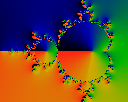Because Z'n has a high 'rotation', it is handier to deal with the normalized vector Pn = Z'n/Zn Here we graph the phase phi=arctan (Re Pn / Im Pn). Note that not all buds are alike: the interior of some buds see a full rotation of the phase by 360 degrees; others do not.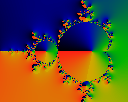Very curiously, this seems to depend on the factorization of the loop termination count. When the loop termination count is prime, none of the buds rotate; when it is a product of many small (repeated) factors, most buds rotate. For example, in this image, most buds rotate because iteration was stopped at 1260=4*9*5*7,whereas this image stopped at 1259 (which is a prime number) and none of the buds rotate.The phase of the second normalized derivative Qn = Z''n/Zn where Z''n+1 = 2 * (Zn * Z''n + Z'2n)

Modulus of second derivative.

Iterated to a high order (N=4153) before stopping.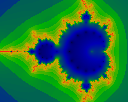Same image as above, but iterated to only N=19. Note the presence of 'zeros' (black dots) on the interior, which are missing from the high-iteration image.As above, but iterated to N=18. Note that this image contains one less black dot on the belt.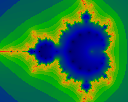Iterated to N=17. Notice how the images generated with prime N are subtly different than those with composite iteration counts.Iterated to N=16. Note that 18 is divisible by 3, while 16 is not: thus the large bud has a green dot in the middle, but the next-smaller one doesn't (unlike the N=18 case).

The second derivative is handy for exhibiting another phenomenon that is hinted at elsewhere in this page, but otherwise difficult to exhibit. This image is created quite artificially: the interior color is recorded only if the modulus of Qn = Z''n/Zn is less than one for some n. In other words, we record the color only if Qn is near a zero. Thus we see a simultaneous depiction of the zero's of the various Qn for various n. These seem to go to a hyperbolic-like limit-circle.

In this image, black is zero, moving through blue, green, yellow to a red of 1.0.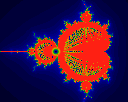Note the Moire patterning make it difficult to discern where the actual limit is. To eliminate this, this image was iterated only to N=50, and so we can see the limit-circle at 50 dots.

The 'distance estimator' is the inverse infinitesimal flow of the iteration number. It gets this name because it provides a rough estimate of how far away an exterior point is from the boundary of the M-Set. The smooth (real-valued) iteration count is given by mu = n+1-log(log(|z|))/log2, as demonstrated in the Escape Theory Room (which is, in turn, the logarithm of the Douady-Hubbard potential). Taking the derivative of mu w.r.t. c we get dmu/dc = d|z|/dc / (|zl log |z| log 2). The 'distance estimator' is one over this quantity.

This first picture shows the iteration to n=9. (We've dropped the factor of log 2).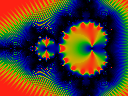Iterate to n=19.Iterate to n=49.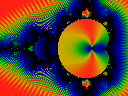Iterate to n=2520=8*9*5*7 a product of small primes. Oddly, the n=2 bulb is unlit, but n=3 and so on light up.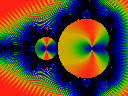Iterate to n=2519 which is a prime number. Oddly, the n=2 bud lights up, the none of the other buds do. Thus, the counting rules here seem to be a bit different than one might expect ...

Don't confuse the absolute value of the derivative with the derivative of the absolute value.

This image shows |z| / |z'|.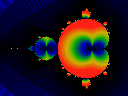This image shows |z| / |z|'.

Copyright (c) 1997, 2000 Linas Vepstas